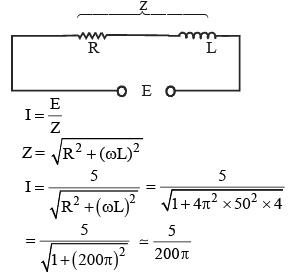Courses

In an LR circuit f = 50 Hz, L = 2 H, E = 5 volts, R = 1 Ω then energy stored in inductor isa)50 Jb)25 Jc)100 Jd)None of theseCorrect answer is option 'D'. Can you explain this answer?

Related TestKamna Science Academy Jul 28, 2021
L = 2 H, E = 5 volts, R = 1ΩL = 2 H, E = 5 volts, R = 1Ω

Top Courses for JEERelated ContentEnergy Stored in CapacitorsDoc | 6 PagesEnergy Stored in CapacitorVideo | 09:34 minEnergy Stored in a CapacitorDoc | 5 Pages